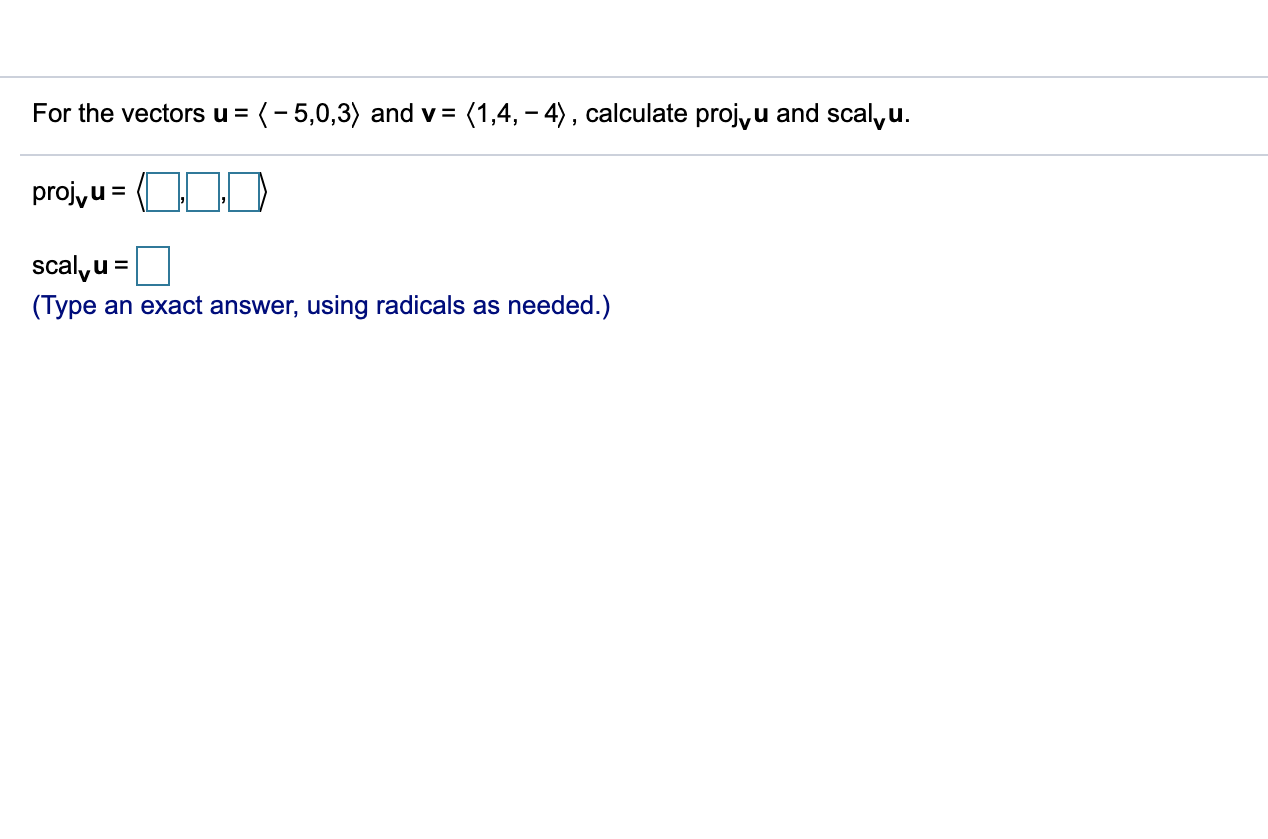# For the vectors u =(-5,0,3) and v= (1,4,-4), calculate projyu and scalyu.projyu=scal,u(Туреan exact answer, using radicals as needed.)

Question
3 viewshelp_outlineImage TranscriptioncloseFor the vectors u = (-5,0,3) and v= (1,4,-4), calculate projyu and scalyu. projyu = scal,u (Туре an exact answer, using radicals as needed.) fullscreen
check_circle

Step 1

The given vectors are u=(-5,0,3) and v=(1,4,-4)

Step 2

To calculate the value of projvu and scalvu

i.e. To find the vector projection of u onto v and scalar projection of u onto v.

Step 3

The given vectors can be written in...

### Want to see the full answer?

See Solution

#### Want to see this answer and more?

Solutions are written by subject experts who are available 24/7. Questions are typically answered within 1 hour.*

See Solution
*Response times may vary by subject and question.
Tagged in

### Calculus